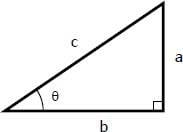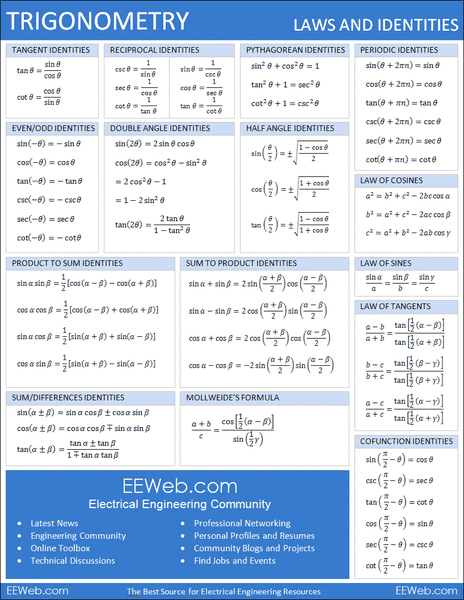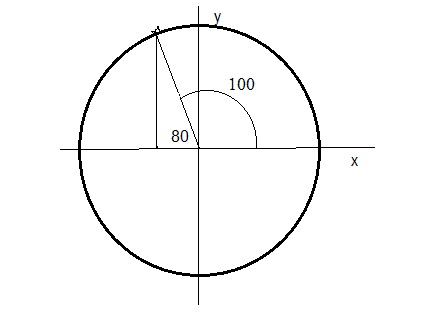# Sin, cos, tan meaning?

I am eager to learn trigonometry.
I have to be introduced to terms such as-sin,cos,tan,cosec etc.
The internet"s explanation is going over my head.
Can someone make them understand to me individually with the meaning of titha.(I cannot show its symbol , as it is not on the keyboard.)
I will grateful to all of them who will help me.

Homework Helper
Gold Member
2020 Award
If you begin with a certain angle ## \theta ##, you can construct a right triangle (90 degrees for the second angle.) The cosine, ## cos(\theta) ##, is the ratio of one side (that goes to the 90 degree point) to the hypotenuse. (The longest side of the triangle=opposite the 90 degree angle.) The sine, ## sin(\theta) ## is the ratio of the side opposite ## \theta ## to the hypotenuse. Knowing the cosine and sine of angles allows for many measurements and calculations on various triangles. The law of sines, ## \frac{sin(a)}{A}=\frac{sin(b)}{B} ## and law of cosines, ## C^2=A^2+B^2-2AB \, cos(c) ## are also very useful in these calculations. (Note: The law of sines and cosines both apply to any type of triangle and not simply to right triangles.)

Last edited:
•Tyler6 and John Dalton
Theta is an intimidating word, but all it really represents is an angle in a given triangle. Sin, cos, and tan are measures of relationships in a triangle with the angle theta.Let this be the triangle we're working with, with theta marked. The hypotenuse is the longest side, in this case marked "C". Sin(theta) is defined as the opposite side (to theta) over hypoteneuse, which in this case would be a/c. Cos is defined as adjacent side over hypoteneuse, or b/c. Tangent is opposite over adjacent, so a/b. Cosec, sec, and cotan are defined as the reciprocal of sin, cos, and tan respectively. You can see that using the fact of pythagoreans theorem, you can use it to prove that sin^2(theta)+cos^2(theta)=1, and this will lead to many other identities you can find. Trig is full of lots of little identities like this, I suggest you try and find more

•alghe, John Dalton and Charles Link
Appreciate all the guidance you have provided me.I was not first initially understanding relation between the theetha and that of Sin,cos,tan .
Now I understood it is an angular unit and is associated with the hypotenuse of the triangle.
Thanks to all.

If you begin with a certain angle ## \theta ##, you can construct a right triangle (90 degrees for the second angle.) The cosine, ## cos(\theta) ##, is the ratio of one side (that goes to the 90 degree point) to the hypotenuse. (The longest side of the triangle=opposite the 90 degree angle.) The sine, ## sin(\theta) ## is the ratio of the side opposite ## \theta ## to the hypotenuse. Knowing the cosine and sine of angles allows for many measurements and calculations on various triangles. The law of sines, ## \frac{sin(a)}{A}=\frac{sin(b)}{B} ## and law of cosines, ## C^2=A^2+B^2-2AB \, cos(c) ## are also very useful in these calculations. (Note: The law of sines and cosines both apply to any type of triangle and not simply to right triangles.)
Superb,informative and precise explanation

•http://ncert.nic.in/textbook/textbook.htm?kemh1=0-16

:)
Absolutely fantastic "rosekidcute"
I was looking out for something like this.

Mark44
Mentor
Can someone make them understand to me individually with the meaning of titha.(I cannot show its symbol , as it is not on the keyboard.)
I think you mean ##\theta##, the lower-case Greek letter "theta". Even though it's not on my keyboard, either, I can use LaTeX to get it, like this: ##\theta##. In addition, many Greek letters are also avaiable as symbols under the ##\Sigma## menu along the top of the text input window.

For historical reasons, Greek letters are used in trigonometry, primarily ##\theta##, ##\alpha##, and ##\beta## (theta, alpha, and beta).

I think you mean θθ\theta, the lower-case Greek letter "theta". Even though it's not on my keyboard, either, I can use LaTeX to get it, like this: ##\theta##. In addition, many Greek letters are also avaiable as symbols under the ΣΣ\Sigma menu along the top of the text input window.
Thanks Mark for this helpful fact,I hope I will use them in my posts from the very next time.☺
Appreciate it!

No problem john dalton , because some time ago i have been searching for a book with everything trigonometry in it toohope this helps too :)

•John Dalton
jbriggs444
Homework Helper
Some folks use the mnemonic "sohcahtoa" meaning "sine = opposite/hypotenuse, cosine=adjacent/hypotenuse, tangent = opposite/adjacent"

You can memorize sohcahtoa or other tricks that people use to get through the next trig test. However, if you ever actually want to use trig, you need to understand the relationships that sine and cosine values have with as you travel along their domain.

One thing that always confused me when learning trig was that the teachers always described it as being all about triangles. And then they presented me with the unit circle and told me that it was also about trig. Then they showed me sine and cosine waves and said "this is trig too". There was no continuity in the way that trig was taught to me, until I was taught by an electrical engineer, who helped me see that sine and cosine are circular functions, just looking at the point on the circle from a different angle (90 degrees apart).

Watch this video :

Watch this video as well

This one goes more in-depth

This video is rather comprehensive

•jerromyjon and alghe
mathwonk
Homework Helper
2020 Award
It is unfortunate today that we have long ago abandoned the teaching of Euclid. The quantity that we now call cosine first appeared in a real sense, but without the name, in the propositions 12 and 13 of Book 2 of Euclid, as generalizations of the Pythagorean theorem. These generalizations are known today as the law of cosines. Namely in a triangle with sides of length A,B,C opposite the angles a,b,c respectively, we have the formula C^2 = A^2 +B^2 -2ABcos(c). The case where c is a right angle and cos(c) = 0 is Pythagoras. In particular angle c is the angle whose sides are A and B.

In Euclid's statement he gives the quantity ABcos(c) as the area of a rectangle whose sides are A and the segment of length x along A obtained by dropping a perpendicular from the end of the side B away from angle c. Referring to the right angle formed by this perpendicular, and by B, and by the segment x cut from A by the perpendicular, by the triangle definition of cosine we have cos(c) = x/B, or x = Bcos(c). Thus the cosine function is a name for the function whose value determines the length of the projection of one side of an angle onto the other side of that angle. (I hope I have corrected my misprints.) (The length of the perpendicular itself is Bsin(c).)

As for the interpretation using circles, Euclid's whole approach to geometry is to use triangles to study circles and vice versa. In cartesian coordinates, the length of the two projections of an angle inscribed in the arc t of the unit circle, onto the two axes, are called cos(t) and sin(t) respectively.

This is my favorite point of view, and the original one. I hope this helps someone.

Last edited:
•alghe, RogueOne and Buffu
rude man
Homework Helper
Gold Member
Surprised no one has addressed the trig functions for angles > 90 degrees. In high school we were just given their definitions without, as I recall, any particular concomitant rationale. I never bothered to figure them out back then & I still haven't!

Mark44
Mentor
Surprised no one has addressed the trig functions for angles > 90 degrees.
@RogueOne posted several videos of trig functions and the unit circle, where the angle/argument is not limited to just acute angles.
rude man said:
In high school we were just given their definitions without, as I recall, any particular concomitant rationale. I never bothered to figure them out back then & I still haven't!
I can't say for sure, since it has been really a long time since I was in high school, but I think we learned the trig functions as defined in the unit circle, not just for angles in a right triangle.

rude man
Homework Helper
Gold Member
@RogueOne posted several videos of trig functions and the unit circle, where the angle/argument is not limited to just acute angles.

I can't say for sure, since it has been really a long time since I was in high school, but I think we learned the trig functions as defined in the unit circle, not just for angles in a right triangle.
How does the "unit circle" provide the rationale for saying sin 100 = sin 80? Why is sin 80 = sin (180 - 80)?
You can't say "sine = opposite over hypotenuse" when the angle exceeds 90 deg!
Etc.

Mark44
Mentor
QUOTE="rude man, post: 5782708, member: 350494"]How does the "unit circle" provide the rationale for saying sin 100 = sin 80? Why is sin 80 = sin (180 - 80)?
You can't say "sine = opposite over hypotenuse" when the angle exceeds 90 deg!
[/QUOTE]Your two equations sin(100°) = sin(80°) and sin(80°) = sin(180° - 80°) are saying exactly the same thing, since 180° - 80° = 100°.
As to why sin(100°) = sin(80°), the cosine and sine of an angle are defined as, respectively, the x and y coordinates on the unit circle for an angle θ[ whose initial side is along the positive x-axis, and whose terminal side extends from the center of the circle to unit circle. (x, y) = (cos θ, sin θ) are the coordinates of the point on the terminal side that intersects the unit circle.

The angle 100° has a terminal side in the second quadrant. If you drop a vertical line segment down to the x-axis, you get the reference triangle (a right triangle) as shown in my drawing here.The acute angle at the origin for this reference triangle is 80°. By symmetry it's easy to see that the triangle I've drawn in the second quadrant can be flipped across the y-axis to get a right triangle in the first quadrant. Clearly sin(80°) is equal to sin(100°) by this symmetry - both triangles have exactly the same altitude, and both have the same hypotenuse (= 1 unit).

It shouldn't be too much of a stretch to see that the magnitudes of the bases of the two triangles are the same, but opposite in sign, so cos(100°) = - cos(80°).

mathwonk
Homework Helper
2020 Award
in post #14 i referred to the law of cosines proved in props 12 and 13 of book 2 of euclid. the reason there are two propositions for one law is that they viewed positive and negative numbers as two separate cases then. i.e. when the angle is > 90degress, cos is negative, and when the angle is < 90degrees the cosine is positive. i recommend taking a look at euclid. your question is also interesting in regard to the proof that the two parallelograms on the same base and with the same height can be decomposed into each other. it is much harder when the vertices of the second triangle are both outside the first parallelogram. see page 14, (day 6):

http://alpha.math.uga.edu/~roy/camp2011/10.pdf

rude man
Homework Helper
Gold Member
To allay all faras: I assuredly did learn how to interpret sines and cosines and the other 4 for angles > 90 deg. But that was and is a matter of definition. It remains that 80 is not 100, sorry.

Mark44
Mentor
It remains that 80 is not 100, sorry.
And so what? No one is claiming that 80° = 100°, but the sine of 80° = the sine of 100°. Similarly sin(30°) = sin(390°), even though 30° and 390° are different angles.

Getting away from trig functions, do you have a problem with someone saying that ##(-2)^2 = (2)^2 = 4##, even though ##-2 \ne +2##? It's really the same idea -- the squaring function and all of the trig functions are not one-to-one, so different inputs can have the same output.

rude man
Homework Helper
Gold Member
And so what? No one is claiming that 80° = 100°, but the sine of 80° = the sine of 100°. Similarly sin(30°) = sin(390°), even though 30° and 390° are different angles.

Getting away from trig functions, do you have a problem with someone saying that ##(-2)^2 = (2)^2 = 4##, even though ##-2 \ne +2##? It's really the same idea -- the squaring function and all of the trig functions are not one-to-one, so different inputs can have the same output.
Hey, I know the rules, have for some 60 years - I'm challenging the rationale, not the rules. I do know they work! The only rationale I know is that they work.

Philosophically speaking, the sine of 100 is a noumenon; the sine of 80 is a phenomenon. There is no such thing as "opposite over hypotenuse" for 100 deg. Saying it's the same as "opposite over hypotenuse" for 80 deg. is a copout that happens to work in our algebraic construct, which is it sole raison d'etre.

Mark44
Mentor
Hey, I know the rules, have for some 60 years - I'm challenging the rationale, not the rules. I do know they work! The only rationale I know is that they work.
That's a pretty strong rationale!

In addition, the real definitions of the cosine and sine of an angle are as mentioned -- the x and y coordinates on the unit circle.
rude man said:
Philosophically speaking, the sine of 100 is a noumenon; the sine of 80 is a phenomenon.
There is no such thing as "opposite over hypotenuse" for 100 deg. Saying it's the same as "opposite over hypotenuse" for 80 deg. is a copout that happens to work in our algebraic construct, which is it sole raison d'etre.
The thing is, in any context more advanced than high school trig, the sine isn't defined as "opposite over hypotenuse." I don't see why sin(100°) is any less a phenomenon than sin(80°) (I had to look up noumenon). If you understand the trig functions in terms of the unit circle, the evidence that the sines of these two angles are equal is easily discernible.

rude man
Homework Helper
Gold Member
That's a pretty strong rationale!